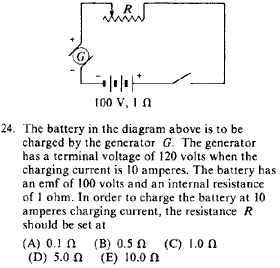GR 8677927796770177 | # Login | Register

GR8677 #24
Problem
 GREPhysics.NET Official Solution Alternate SolutionsElectromagnetism}Circuits

Apply Kirchhoff's Loop Law: , where is the voltage of the generator and is the voltage of the battery, and the extra is the internal resistance. in the problem, and thus .Alternate Solutions
 secretempire12013-03-18 10:58:28 The easiest way I can think of is the following: Look at the positive side of the generator, and the positive side of the battery. In between, there is a 20 V drop across two resistors. Using Ohm's law, I = 10A = V/R = 20 V / 1+R. Solve for R, it's that simple.Reply to this commentcasseverhart13
2019-10-11 06:38:36
Thanks, very nice problem. https://www.pressurewashingcapecoral.comfredluis
2019-08-09 04:24:48
Note that if the fields were perpendicular to each other the electron would drift in a helical pattern as in choice (C). tree removaljoshuaprice153
2019-08-09 01:54:59
I’m happy I located this blog! From time to time, students want to cognitive the keys of productive literary essays composing. Your first-class knowledge about this good post can become a proper basis for such people. nice one! makeup artist Orlandoblacksheep
2017-09-21 23:33:20
You certainly could do this using Kirchoff\'s Loop Rule, but I just took the approach you did of writing an equation based on what\'s happening. What\'s important to realize is that the battery in this problem is being *charged,* it is not a source of emf; the generator is the source. Thus the voltage drop due to the battery\'s internal resistance is added to the emf, as is the voltage drop across the resistor, all of which must be supplied by the generator. My equation looked like this: (100 volts) + (10 amps)(1 ohm) + (10 amps)(R) = 120 volts. Solve for R to get 1 ohm.richard7893
2015-05-05 08:21:24
Don't understand the R+1 part. Here's my loop:

-120V + (100V - 10A(1 ohm)) - 10A(R)=0

R=3 ohm , not a choice. Can someone let me know where I went wrong?
 Jdogzz2015-10-22 18:01:38 Apologies for the poor formatting, I have no idea why it went so horribly...secretempire1
2013-03-18 10:58:28
The easiest way I can think of is the following:

Look at the positive side of the generator, and the positive side of the battery. In between, there is a 20 V drop across two resistors.

Using Ohm's law, I = 10A = V/R = 20 V / 1+R.

Solve for R, it's that simple.pauria
2010-07-02 23:03:17
Sorry, forgot to flag the below as typo.pauria
2010-07-02 23:02:32
I don't know what kind of arithmetic is going on here but the answer is R=1, not 1/2.

Just check the answer table at the top; it's C.
 pam d2011-09-23 20:57:55 Yosun has the answer as 1 ohm, that 1/2 you're seeing is just a 1 with capital omega.angiep
2005-11-11 14:32:45
typo: I = 10 amperes
 yosun2005-11-11 20:39:02 angiep: thanks for the typo-alert; the typo has been corrected.LaTeX syntax supported through dollar sign wrappers $, ex.,$\alpha^2_0$produces . type this... to get...$\int_0^\infty\partial\Rightarrow\ddot{x},\dot{x}\sqrt{z}\langle my \rangle\left( abacadabra \right)_{me}\vec{E}\frac{a}{b}\$